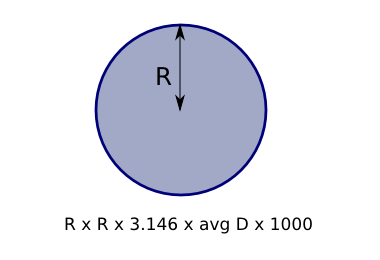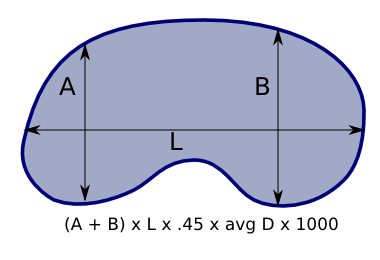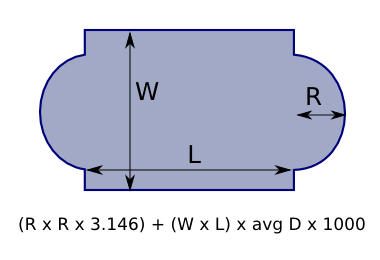0

by Tim Hoffman May 01, 2019 1 min read

Here are some general guides on calculating pond volumes for different pond shapes. Most results will always be approximations as sides of ponds are typically not vertical, the bottom pond isn't flat or the calculation is fundamentally an approximate.  We have chosen to present the formula as calculating the volume in litres, as all of the ponds on this site are published with a litres per hour flow rate. If you have any problems working out your pond volume please contact us and we will try help.

Here is an example using the rectangle pond.  If you pond is 2.5m long and 1.2m wide and about 40cm deep, you would then do the following using the  formula (W x L x average depth x 1000)   1.2 x 2.5 x 0.4 x 1000 =   1200 litres.When calculating the volume of a cylinder, R refers to the radius.For elliptical cylinders your using the shortest (A) and longest (B) radii of the elipse.The formula for kydney shaped ponds is very approximate.  I would generally round up to the nearest 500 lites when calculating the volume.With rectangular ponds with round ends you are effectively calculating the depth of two ponds, a round and rectangular pond added together.